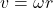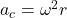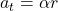## Consider an object on a rotating disk a distance r from its center, held in place on the disk by static friction. Which of the following sta

Question

Consider an object on a rotating disk a distance r from its center, held in place on the disk by static friction. Which of the following statements is not true concerning this object:

A) If the angular speed is constant, the object must have constant tangenial speed.
B) If the angular speed is constant, the object is not accelerated
C) The object has a tangenial acceleration only if the disk has an angular acceleration.
D) If the disk has an angular acceleration, the object hasboth a centripetal and a tangenial acceleration
E) The object always has a centripetal acceleration exceptwhen the angular speed is zero

in progress 0
2 months 2021-07-29T21:07:31+00:00 1 Answers 2 views 0

A) False

B) False

C) True

D) True

E) True

Explanation:

A) The formula for tangential speed v in term of angular speed ω and radius of rotation r isSo if the angular speed is constant and 0, the tangential speed is also 0. A) is false

B) False because of the centripetal acceleration:C) True because of the formula for tangential acceleration in term of angular acceleration α isD) True because same as D), if it has angular acceleration, it would have a tangential acceleration. Also from B) the centripetal acceleration will come with time as soon as angular speed is generated by angular acceleration.

E) True and same explanation as from B)